Next: Vector Line Integrals Up: Vectors and Vector Fields Previous: Vector Calculus

# Line Integrals

Consider a two-dimensional functionthat is defined for alland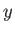. What is meant by the integral of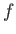along a given curve joining the pointsandin the-plane? Well, we first draw outas a function of length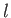along the path. (See Figure A.13.) The integral is then simply given by(A.69)

where.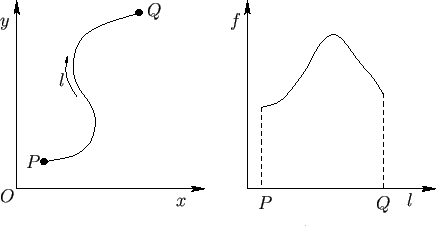For example, consider the integral of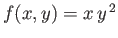betweenandalong the two routes indicated in Figure A.14. Along route 1, we have, so. Thus,(A.70)

The integration along route 2 gives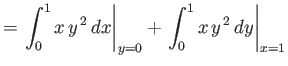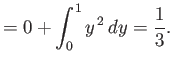(A.71)

Note that the integral depends on the route taken between the initial and final points.The most common type of line integral is that in which the contributions fromand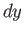are evaluated separately, rather that through the path-length element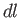: that is,(A.72)

For example, consider the integral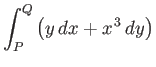(A.73)

along the two routes indicated in Figure A.15. Along route 1, we haveand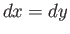, so(A.74)

Along route 2,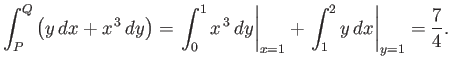(A.75)

Again, the integral depends on the path of integration.Suppose that we have a line integral that does not depend on the path of integration. It follows that(A.76)

for some function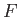. Given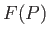for some pointin the-plane,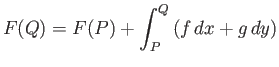(A.77)

defines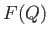for all other points in the plane. We can then draw a contour map of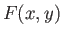. The line integral between pointsandis simply the change in height in the contour map between these two points: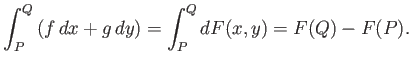(A.78)

Thus,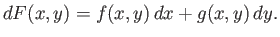(A.79)

For instance, if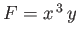then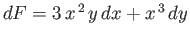and(A.80)

is independent of the path of integration.

It is clear that there are two distinct types of line integral--those that depend only on their endpoints and not on the path of integration, and those that depend both on their endpoints and the integration path. Later on, we shall learn how to distinguish between these two types. (See Section A.18.)Next: Vector Line Integrals Up: Vectors and Vector Fields Previous: Vector Calculus
Richard Fitzpatrick 2016-03-31